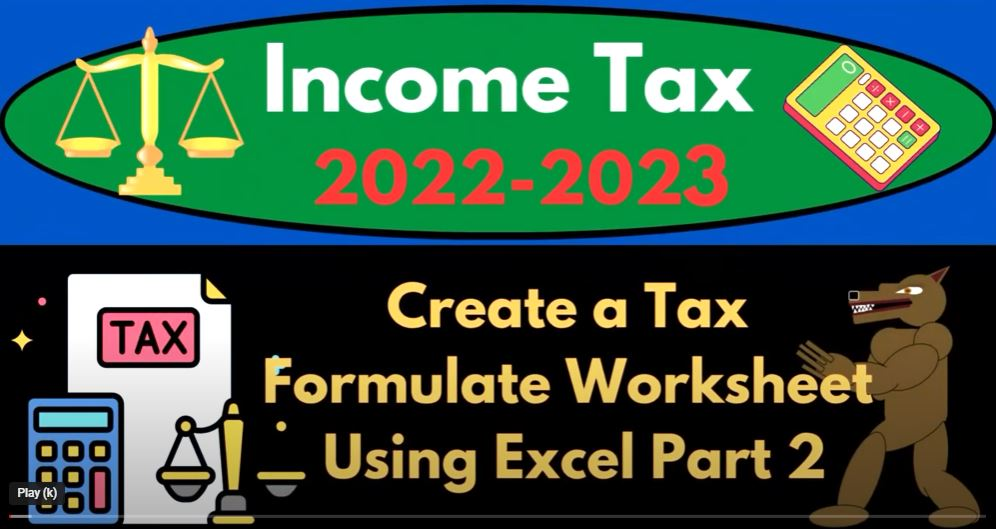## Create a Tax Formulate Worksheet Using Excel Part 2 1505 Income Tax Preparation 2022 – 2023

Great, let’s continue working on the tax formula worksheet in Excel. In our previous session, we outlined the income tax formula, so now let’s focus on some of the key components of the formula and how we can incorporate them into our Excel worksheet.

1. Taxable Income: This is the amount of income that is subject to federal income tax after accounting for deductions and exemptions. We can calculate taxable income by subtracting deductions and exemptions from the total income. In Excel, we can use the formula “=Total Income – Deductions – Exemptions” to calculate taxable income.
2. Tax Brackets: The income tax system uses a progressive tax structure, which means that as income increases, the tax rate also increases. There are different tax brackets with different tax rates, and the amount of income that falls into each bracket determines the tax rate that applies to that portion of income. We can use Excel’s VLOOKUP function to determine the tax rate for each bracket based on the taxable income amount.
3. Tax Credits: Tax credits can help reduce the amount of tax owed. There are different types of tax credits, such as the child tax credit, education credits, and energy credits. We can use Excel’s SUM function to calculate the total amount of tax credits.
4. Alternative Minimum Tax (AMT): The AMT is an alternative way of calculating tax liability that limits certain deductions and exemptions. Taxpayers are required to pay the higher of the regular income tax or the AMT. We can use an IF statement in Excel to check if the taxpayer is subject to the AMT and calculate the AMT if necessary.

Putting all of these components together, we can create an Excel formula that calculates federal income tax liability:

=IF(Taxable Income<=0,0,VLOOKUP(Taxable Income, Tax Brackets, 2, TRUE)Taxable Income-SUM(Tax Credits)-IF(Taxable Income>AMT Threshold, VLOOKUP(Taxable Income, AMT Rates, 2, TRUE)(Taxable Income-AMT Exemption), 0))

Note that this is just an example formula and you may need to adjust it based on your specific tax situation. Also, make sure to double check your calculations and consult with a tax professional if you have any questions.

In this tutorial, we will create a color-coded tax formula in Excel, which will make it easier to understand the different types of data inputs and calculations involved in tax preparation.

First, we will create a table with the different tax brackets and rates, which will be used in our tax formula. Then, we will create a new worksheet for our tax formula.

In the new worksheet, we will start by inputting our income, which we will make green to indicate positive numbers. Then, we will input our itemized deductions, which will come from another tab and will be blue to indicate that we need to do something to it. Next, we will input our standard deductions, which will come from a table below and will also be blue.

Our next input will be our other qualified deductions, which may come directly from a Schedule C or another worksheet, and will also be blue. This will give us our taxable income, which will be calculated using a formula, so we will not need to input any data in this cell.

Next, we will input our estimated tax payments, which will come from another tab, and will also be blue. This will be subtracted from our tax liability to give us our total tax. This will also be a formula, so we will not need to input any data in this cell.

Our final input will be our payments and other credits, which will also come from another tab, and will not be blue as we will not need to manually input any data in this cell.

We will format our inputs by making our income green, our deductions red to indicate negative numbers, and our tax rate will be left as is. This will help us to distinguish between good and bad inputs.

Lastly, we will color code our tax liability and credits. We will make our tax liability red to indicate that it is bad, and our credits green to indicate that they are good.

By creating this color-coded tax formula, we can quickly and easily identify the different types of data inputs and calculations involved in tax preparation, which will help us to avoid errors and ensure that we are accurately preparing our taxes.